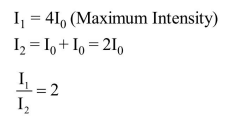Deepak Scored 45->99%ile with Bounce Back Crack Course. You can do it too!

# In a Young's double slit experiment, the two slits act as coherent sources of waves of equal amplitude A and wavelength

Question:

In a Young's double slit experiment, the two slits act as coherent sources of waves of equal amplitude A and wavelength $\lambda$. In another experiment with the same arrangement the two slits are made to act as incoherent sources of waves of same amplitude and wavelength. If the intensity at the middle point of the screen in the first case is $\mathrm{I}_{1}$ and in the second case $\mathrm{I}_{2}$, then the ratio $\frac{\mathrm{I}_{1}}{\mathrm{I}_{2}}$ is :-

1. 4

2. 2

3. 1

4. 0.5

Correct Option:

Solution: